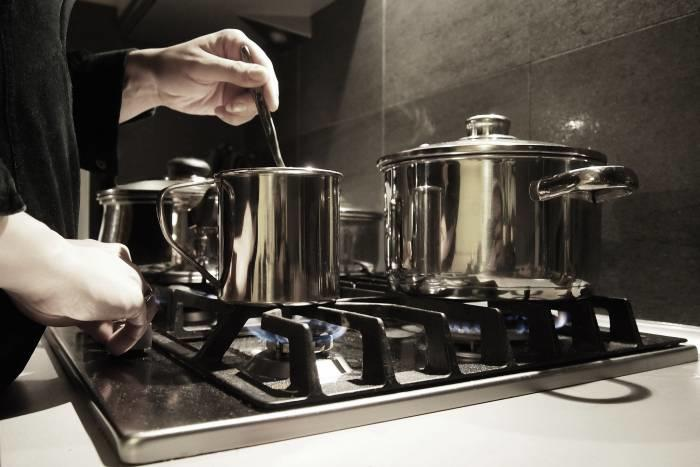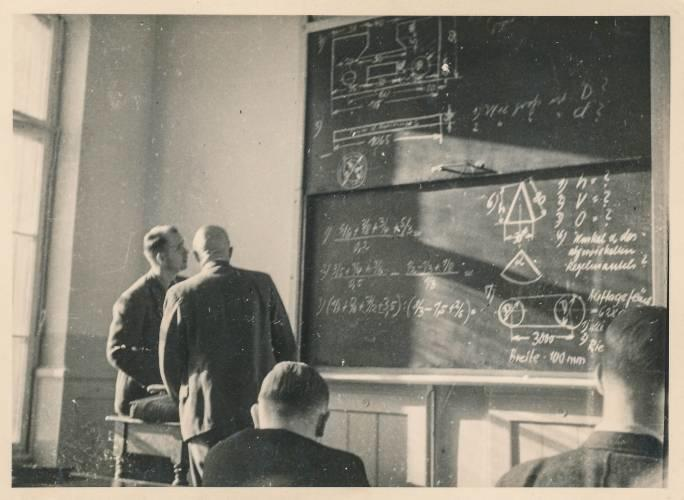Why Learn Maths?

Like many of us, you may not be too keen on listening to your maths teacher and your maths education may not be filled with inspiration.

Apart from the fact that the National Curriculum and the school system impose maths lessons on us, what good reasons are there for all that maths homework? Believe it or not, maths isn't just for the classroom.

There numerous concrete applications in our everyday life. In spite of those who might claim that "Maths, fractions, decimals and all that: It's useless!" there are in fact many examples to be found of in everyday life.

Overall, maths and maths concepts actually helps us to understand the world around us.

It doesn't need to be overly challenging concepts! So, how can basic math (addition and subtraction, times tables, algebra and geometry) be used to solve puzzles?

## What is Maths Good for in Everyday life?

Maths expressions are present in all aspects of our lives, from our most tender years right up until the end of our lives.Cooking: an area ripe for the application of maths knowledge! (Source: Flickr.com - Damian Siwiaszczyk)

Learning maths is, therefore, an essential part of everyone's life.

In following the school curriculum, maths classes lead us through learning trigonometry, algebra questions, arithmetic, Thales' theorem, calculus, linear equations, multiplication and division, decimals, integers, quadratic equations and more.

But in what practical situations, apart from maths lessons, quizzes and exams can we apply all this knowledge?

Here are some examples:

• Doing the shopping and calculating a budget for groceries,
• Buying a house or apartment: Calculating the rates at which money is borrowed, and repayments.
• Cooking: Measuring weights, temperatures and mixtures.
• DIY: Measuring distances, angles (sine, cosine, right angles), weights and volumes,
• Getting around: Calculating distances, fuel consumption
• Gambling: Poker, blackjack, bridge.

All those maths lessons spent learning multiplication tables, square roots, long division and geometry also have a place in one's professional life, especially in sales, accounting, architecture or IT.

Simple exercises such as this can help relieve math anxiety, as you begin to understand the importance of maths and number sense in your daily life. If that's not enough, consider taking lessons with a math tutor to help your understanding of numbers.Arun
Maths Teacher4.67 (6) ₹750/h
1st class FREE!Surya
Maths Teacher5.00 (20) ₹700/h
1st class FREE!Sarbartha
Maths Teacher5.00 (4) ₹150/h
1st class FREE!Ayushi
Maths Teacher5.00 (10) ₹600/h
1st class FREE!Vilsy
Maths Teacher5.00 (5) ₹500/h
1st class FREE!Sahil
Maths Teacher4.86 (7) ₹120/h
1st class FREE!N kumar
Maths Teacher5.00 (3) ₹1,599/h
1st class FREE!Arun
Maths Teacher5.00 (1) ₹500/h
1st class FREE!

## Why is Maths Important? To Understand the World better

Mathematics is of great social importance. So, therefore, are maths teachers.

As a student, teachers guide us, from nursery school to primary school, through to year 11 maths, in better understanding the codes that comprise the world around us. It is a world that rests heavily on the assumption that 1 + 1 = 2.

Many professions which provide valued services, including meteorology, pharmaceutical development, medical imaging and data management are greatly influenced by geometry, arithmetic or algebra.To understand the laws that govern our planet and its universe: turn to maths (Source: Flickr.com - Kevin Gill)

The study of maths, therefore, is also the study of the world. The solar system, weather and climate, and how could we neglect to mention cartography, which has been of huge importance for decades?

Here's how all those maths exercises, encountered since primary school in textbooks and worksheets, can lead to such a great impact on the development of the world.

On a more personal level, mathematics is always present at home. The layout of your living space and its arrangement involves conceptualising interiors in 2D, then in 3D, to understand the geometry of a space.

Finally, learning maths also allows you to understand various health issues, from a simple medical record to imaging and ultrasound. It is thanks to the teaching of maths and to the National Curriculum that we have learnt to understand and better manage our own bodies.

## 5 Maths Puzzles that still have us Baffled

Before attempting such advanced applications of maths, we need to first learn to love the topic. This can sometimes be achieved through a maths teacher who happens to be particularly talented, or to lessons that are especially entertaining.

As far as maths resources are concerned, there are some that particularly pique the curiosity of a maths student, whether at university, high school, college or during private maths tuition: Mysteries!

Some of the great mysteries of maths have already been solved by great minds. Others still remain to be decoded, including:

• The Pyramids of Egypt
• The Sphinx
• The Iron Pillar of Delhi
• The mathematical genius of some of the greats: Einstein, Newton, Poincaré, De Vinci, Archimedes, Hawking

Learning maths is not that simple, however. It is effectively a language in its own right, with its own special vocabulary, hundreds of formulas to remember, and numerous derivative disciplines in the form of other scientific subjects: Physics chemistry, biology, life sciences, technology, engineering, and so on.Could you solve one of these great maths puzzles? (Source: Flickr.com - Hans Schule)

One would need a deep understanding of many areas of maths, in order to be able to solve one of the following famous maths problems:

## Mathematical Equations Changed the Course of History

Learning maths at school elevates the student to a high level of knowledge. Whether you are a good student or a bad one, you are sure to have gone through the study of mental arithmetic, solving maths problems and perhaps even division word problems.

But among all that is taught, certain mathematical concepts and equations have gained a place in maths history as indispensable.

This is particularly the case for the following ten, which you may have heard about from your physics or maths tutor:

## Learning Maths Can Be A Secret Weapon in Poker

After pure and unadulterated mathematics, how about we look at maths through the lens of money and gambling? Can your maths classes be applied to poker, for example?

Poker is not just a game of chance, and is in fact intimately linked to maths – another good reason to learn it! Poker is characterised by a multitude of possible card combinations, but also by learning to keep a cool head and manage one's emotions.

The other great ability to possess to be a good poker player: to understand probability!

In this, maths is essential.

The goal: To know how often certain combinations of cards will be drawn, according to those in your hand and those on the table. Probabilities, however, are not the only mathematical tools used.

We can talk about "outs", for example, or pot odds.

And finally, you need to have a good head for mental arithmetic to make good use of maths in poker!

Hopefully these real world examples of the benefits of learning maths have given you some good motivation to try harder to learn maths!

Need a teacher Maths ?0.00/5, 0 votesLoading...